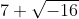## Polar Form

Convert the following into polar form:Hint
Complex numbers can be written in both rectangular and polar form. For rectangular form, a complex number is represented by both its real and imaginary components:
$$z=a+jb$$$where $$a$$ is the real component, $$b$$ is the imaginary component, and $$j=\sqrt{-1}$$ which is often expressed as $$i=\sqrt{-1}$$ . Hint 2 Polar form is $$z=c\: \angle \: \theta$$$
where $$c=\sqrt{a^2+b^2}$$ (the vector’s length) and $$\theta=tan^{-1}(b/a)$$ . Note: $$a=c\:cos(\theta)$$ and $$b=c\:sin(\theta)$$ .
Complex numbers can be written in both rectangular and polar form. For rectangular form, a complex number is represented by both its real and imaginary components:
$$z=a+jb$$$where $$a$$ is the real component, $$b$$ is the imaginary component, and $$j=\sqrt{-1}$$ which is often expressed as $$i=\sqrt{-1}$$ . Thus, our rectangular coordinate form can be rewritten as: $$7+\sqrt{-1}\sqrt{16}\to 7+4i$$$
where $$a=7$$ and $$b=4$$ .
Polar form is:
$$z=c\: \angle \: \theta$$$where $$c=\sqrt{a^2+b^2}$$ (the vector’s length) and $$\theta=tan^{-1}(b/a)$$ . Note: $$a=c\:cos(\theta)$$ and $$b=c\:sin(\theta)$$ Therefore, $$c=\sqrt{7^2+4^2}=\sqrt{49+16}=\sqrt{65}=8.06$$$
$$\theta=tan^{-1}(\frac{4}{7})=29.7^{\circ}$$$Finally, the polar form is: $$8.06 \: \angle \: 29.7^{\circ}$$$
$$8.06 \: \angle \: 29.7^{\circ}$$\$